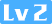徐小夕1049 0 0

## 你将收获

• 链表的概念和应用
• 原生javascript实现一条单向链表
• 原生javascript实现一条个双单向链表
• 链表和数组的对比及优缺点

## 正文

### 2.原生javascript实现一条单向链表

#### 2.1 定义链表结构

``````let Node = function(el) {
this.el = el;
this.next = null;
}``````

``````// 单向链表, 每一个元素都有一个存储元素自身的节点和一个指向下一个元素引用的节点组成
let Node = function(el) {
this.el = el;
this.next = null;
}
let length = 0
let head = null  // 用来存储第一个元素的引用

// 尾部添加元素
this.append = (el) => {};
//插入元素
this.insert = (pos, el) => {};
// 移除指定位置的元素
this.removeAt = (pos) => {};
// 移除指定节点
this.remove = (el) => {};
// 查询节点所在位置
this.indexOf = (el) => {};
// 判断链表是否为空
this.isEmpty = () => {};
// 返回链表长度
this.size = () => {};
// 将链表转化为数组返回
this.toArray = () => {};
}``````

#### 2.2 实现添加节点

``````// 尾部添加元素
this.append = (el) => {
let node = new Node(el),
current;
}else {
while(current.next) {
current = current.next;
}
current.next = node;
}
length++
};``````

#### 2.3 实现插入节点

``````//插入元素
this.insert = (pos, el) => {
if(pos >=0 && pos <= length) {
let node = new Node(el),
previousNode = null,
curIdx = 0;
if(pos === 0) {
node.next = current;
}else {
while(curIdx++ < pos) {
previousNode = current;
current = current.next;
}
node.next = current;
previousNode.next = node;
length++;
return true
}
}else {
return false
}
};  ``````

#### 2.4 根据节点的值查询节点位置

``````// 查询节点所在位置
this.indexOf = (el) => {
let idx = -1,
curIdx = -1,
while(current) {
idx++
if(current.el === el) {
curIdx = idx
break;
}
current = current.next;
}
return curIdx
}; ``````

#### 2.5 移除指定位置的节点

`````` // 移除指定位置的元素
this.removeAt = (pos) => {
// 检测边界条件
if(pos >=0 && pos < length) {
let previousNode = null,
curIdx = 0;
if(pos === 0) {
// 如果pos为第一个元素
}else {
while(curIdx++ < pos) {
previousNode = current;
current = current.next;
}
previousNode.next = current.next;
}
length --;
return current.el
}else {
return null
}
};``````

#### 2.6 移除指定节点

``````  // 移除指定节点
this.remove = (el) => {
let idx = this.indexOf(el);
this.removeAt(idx);
}; ``````

#### 2.7 获取节点长度

``````// 返回链表长度
this.size = () => {
return length
}; ``````

#### 2.8 判断链表是否为空

``````// 返回链表长度
this.size = () => {
return length
};``````

#### 2.9 打印节点

``````// 将链表转化为数组返回
this.toArray = () => {
results = [];
while(current) {
results.push(current.el);
current = current.next;
}
return results
}; ``````

``````let link = new linkedList()
// 添加节点
// 查找节点
// ...``````

### 3.原生javascript实现一条个双单向链表

``````let Node = function(el) {
this.el = el;
this.previous = null;
this.next = null;
}
let length = 0
let head = null  // 用来存储头部元素的引用
let tail = null  // 用来存储尾部元素的引用``````

``````// 双向链表, 每一个元素都有一个存储元素自身的节点和指向上一个元素引用以及下一个元素引用的节点组成
let Node = function(el) {
this.el = el;
this.previous = null;
this.next = null;
}
let length = 0
let head = null  // 用来存储头部元素的引用
let tail = null  // 用来存储尾部元素的引用

// 尾部添加元素
this.append = (el) => {
let node = new Node(el)
}else {
tail.next = node;
node.previous = tail;
}
tail = node;
length++
};
// 插入元素
this.insert = (pos, el) => {
if(pos >=0 && pos < length) {
let node = new Node(el);
if(pos === length - 1) {
// 在尾部插入
node.previous = tail.previous;
node.next = tail;
tail.previous = node;
length++;
return true
}
i = 0;
while(i < pos) {
current = current.next;
i++
}
node.next = current;
node.previous = current.previous;
current.previous.next = node;
current.previous = node;
length ++;
return true
}else {
throw new RangeError(`插入范围有误`)
}
};
// 移除指定位置的元素
this.removeAt = (pos) => {
// 检测边界条件
if(pos < 0 || pos >= length) {
throw new RangeError(`删除范围有误`)
}else {
if(length) {
if(pos === length - 1) {
// 如果删除节点位置为尾节点,直接删除,节省查找时间
let previous = tail.previous;
previous.next = null;
length --;
return tail.el
}else {
previous = null,
next = null,
i = 0;
while(i < pos) {
current = current.next
i++
}
previous = current.previous;
next = current.next;
previous.next = next;
length --;
return current.el
}
}else {
return null
}
}
};
// 移除指定节点
this.remove = (el) => {
let idx = this.indexOf(el);
this.removeAt(idx);
};
// 查询指定位置的链表元素
this.get = (index) => {
if(index < 0 || index >= length) {
return undefined
}else {
if(length) {
if(index === length - 1) {
return tail.el
}
i = 0;
while(i < index) {
current = current.next
i++
}
return current.el
}else {
return undefined
}
}
}
// 查询节点所在位置
this.indexOf = (el) => {
let idx = -1,
curIdx = -1;
while(current) {
idx++
if(current.el === el) {
curIdx = idx;
break;
}
current = current.next;
}
return curIdx
};
// 判断链表是否为空
this.isEmpty = () => {
return length === 0
};
// 返回链表长度
this.size = () => {
return length
};
// 将链表转化为数组返回
this.toArray = () => {
results = [];
while(current) {
results.push(current.el);
current = current.next;
}
return results
};
}``````

• 插入删除性能
• 查询性能
• 内存占用

## 更多推荐

### 相关推荐

《前端实战总结》之使用解释器模式实现获取元素Xpath路径的算法

4K@60智能云台从零到一
![](https://oscimg.oschina.net/oscnet/378c3404-772f-4a7b-8968-a2fd109e61cb.png) 正文字数：6006  阅读时长：9分钟 > 近两年以来，短视频受到越来越多用户的喜爱和追捧，但短视频的制作成本却扼杀了大量内容创作者的热情和动力。LiveVideoStackCon2020北京
Curl之Post Json
curl Post Json \$ curl -i -X POST -H "'Content-type':'application/x-www-form-urlencoded', 'charset':'utf-8', 'Accept': 'text/plain'" -d 'json_data={"a":"aaa","b":"bbb","data
IE和Firefox下event乱谈

JavaScript零基础入门——（一）什么是JavaScript
JavaScript零基础入门——（一）什么是JavaScript ================================= 写在前面： 『Hello，大家好，我是振丹！从这节课开始，我会慢慢的带大家学习JavaScript的基础，至于进阶部分，有机会我也会专门开专题来讲。有做后端同学会说，现在微软的TypeScript开始火起来了，连Angu
JavaScript零基础入门——（十二）JavaScript的定时器
JavaScript零基础入门——（十二）JavaScript的定时器 =================================== 大家好，欢迎回到我们的JavaScript零基础入门。上一节课我们讲了JavaScript中一些常用的DOM操作，这里要补充一个点，上节课讲的table几个常用属性其实是有兼容性问题的，在部分IE浏览器中是不识别的
Node.js学习路线图